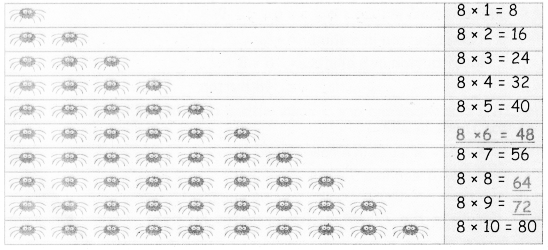Students can download 4th Maths Term 2 Chapter 2 Numbers Ex 2.3 Questions and Answers, Notes, Samacheer Kalvi 4th Maths Guide Pdf helps you to revise the complete Tamilnadu State Board New Syllabus, helps students complete homework assignments and to score high marks in board exams.

## Tamilnadu Samacheer Kalvi 4th Maths Solutions Term 2 Chapter 2 Numbers Ex 2.3

Question 1.
3 × 7 = ______
3 × 7 =   21Question 2.
6 × 7 = ______
6 × 7 =   46

Question 3.
9 × 7 = ______
9 × 7 =   63

Question 4.
A box contains 7 pens. How many pens are 1here in 5 boxes?
Ans.
Number of pen in a box = 7
Number of pens in 5 boxes = 7 × 5
= 35 PensQuestion 5.
One week has 7 days. Calculate the number of days in 10 weeks?